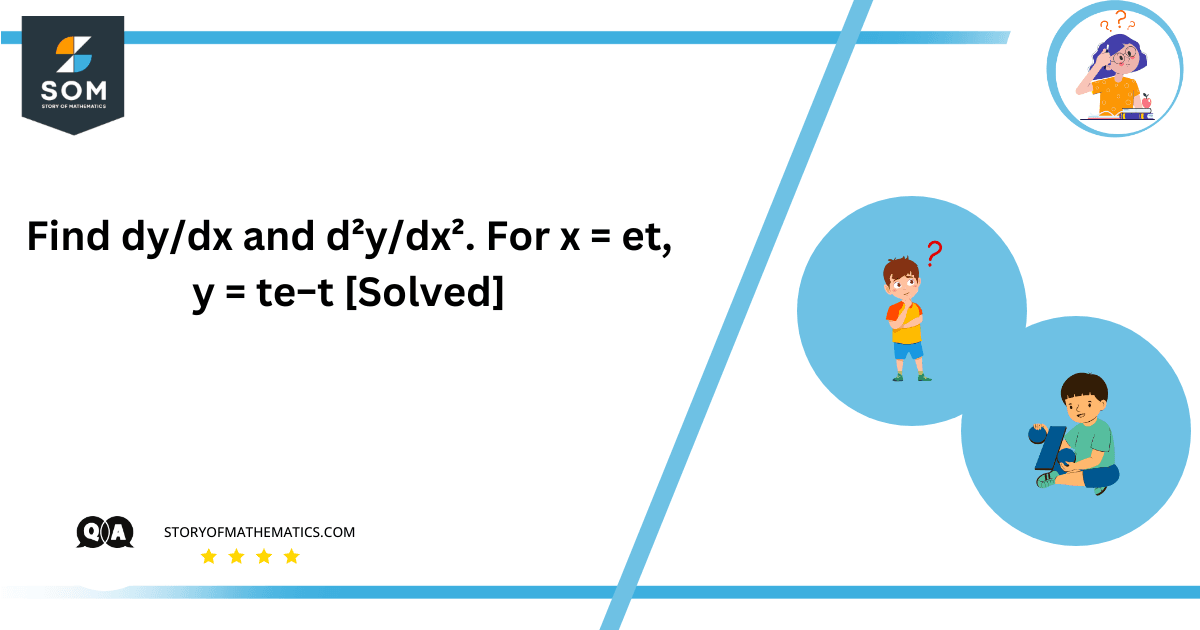# Find dy/dx and d²y/dx². For x = et, y = te−t [Solved]The first derivative dy/dx gives us the rate of change of y with respect to x which is $(e^{(-t)} – t * e^{(-t)})$ / $e^t$ and the second derivative d²y/dx² is [(- $e^{(-t)} + t * e^{(-t)}) * e^t – (e^{(-t)} – t * e^{(-t)}) * e^t] / e^{(2t)}$ = -2.

To find dy/dx and d²y/dx² at x = $e^t$, y = t$e^{(-t)}$, we’ll differentiate y with respect to x using the chain rule. By differentiating y with respect to x using the chain rule, we aim to uncover valuable insights into the slope and curvature of this curve.

First, we need to calculate dy/dt and dx/dt, which will allow us to find dy/dx using the chain rule.

Given: x = $e^t$ and y = t$e^{(-t)}$

dx/dt: To find dx/dt, we differentiate x with respect to t:

dx/dt = d($e^t$)/dt = $e^t$

dy/dt: To find dy/dt, we differentiate y with respect to t:

dy/dt = d(te^(-t))/dt = e^(-t) – t * e^(-t)

Now that we have dx/dt and dy/dt, we can calculate dy/dx:

dy/dx = (dy/dt) / (dx/dt)

= $(e^{(-t)} – t * e^{(-t)})$ / $e^t$

This is the first derivative, which gives us the rate of change of y with respect to x.

Next, let’s find d²y/dx², the second derivative. To do this, we’ll differentiate dy/dx with respect to x:

d²y/dx² = d/dx[$(e^{(-t)} – t * e^{(-t)})$ / $e^t$]

Using the quotient rule and chain rule, we differentiate each term separately:

d/dx$(e^{(-t)} – t * e^{(-t)})$ = – $e^{(-t)} – (-t * e^{(-t)})$ =

= – $e^{(-t)} + t * e^{(-t)} d/dx(e^t)$

= e^t

Now, we can apply the quotient rule:

d²y/dx² = [(- $e^{(-t)} + t * e^{(-t)}) * e^t – (e^{(-t)} – t * e^{(-t)}) * e^t] / (e^t)^2$

Simplifying the expression:

d²y/dx² = [(- $e^{(-t)} + t * e^{(-t)}) * e^t – (e^{(-t)} – t * e^{(-t)}) * e^t] / e^{(2t)}$

Simplify the expression:

d²y/dx² = -2

So, d²y/dx² is a constant value of -2.

## Numerical Result

The first derivative dy/dx is $(e^{(-t)} – t * e^{(-t)})$ / $e^t$ and the second derivative d²y/dx² is -2.

To determine where the curve is concave upward, we need to find where d²y/dx² is positive. Since d²y/dx² is always -2, the curve is never concave upward. Therefore, the curve is concave downward for all values of t.

## Example

Calculate the derivatives for x = $e^t$ and y = t * $e^{(-t)}$ to find dy/dx and d²y/dx² at t = 2

## Solution

First, we’ll find the values of x and y at t = 2:

x = $e^2$

≈7.389

y = 2 * $e^{(-2)}$

≈0.2707

Now, let’s calculate dy/dx at t = 2 using the derivatives we previously derived:

dy/dx = ($e^{(-2)} – 2 * e^{(-2)})/e^2$

≈ (0.1353 – 0.5415)/7.389

≈ -0.0513

Next, let’s find d²y/dx² at t = 2:

d²y/dx² = [$(-e^{(-2)} + 2 * e^{(-2)}) * e^2 – (e^{(-2)} – 2 * e^{(-2)}) * e^2]/e^{(4)}$

≈ [(-0.1353 + 0.5415) * 7.389-(-0.1353 + 0.5415) * 7.389]/54.598

≈ 0